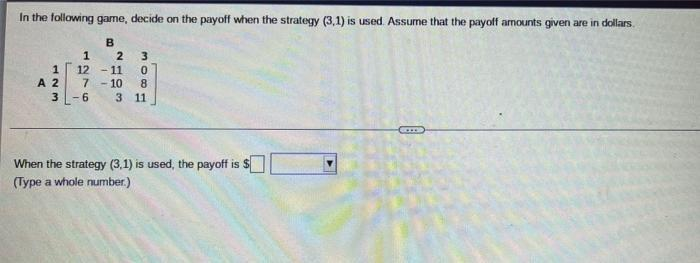Question:

# In the following game, decide on the payoff when the strategy (3,1) is used. Assume that the payoff amounts given are in dollars. 1 2 3 1 [12 -11In the following game, decide on the payoff when the strategy (3,1) is used. Assume that the payoff amounts given are in dollars. 1 2 3 1 [12 -11 0] 2 [7 -10 8] 3[- 6 3 11] When the strategy (3,1) is used, the payoff is \$